# 前言：兼顾效率和准确性

SDD出现之前，主流的CNN目标检测模型分别是Faster R-CNNYOLO，Faster R-CNN作为two-stage的代表，具有state of the art的准确性，但是速度偏慢，做不到实时。YOLO使得目标检测任务one-stage就能完成，在效率上有了明显改善，但是准确性上确差了很多。这就好比“人有悲欢离合，月有阴晴圆缺，此事古难全。”

SSD的论文是《SSD: Single Shot MultiBox Detector》，下面我们就来看一下它具体是如何实现的。

# YOLO实现

## 设计理念

• 从不同的卷积层中分别拉取分支进行检测，不同的卷积层具有多种感受野和语义信息
• 用卷积层代替全连接层和reshape层，用卷积核的数量控制输出特征图的通道数
• 借鉴Faster R-CNN，进入用先验atchor box，并对每一个box都预测类别

## 网络结构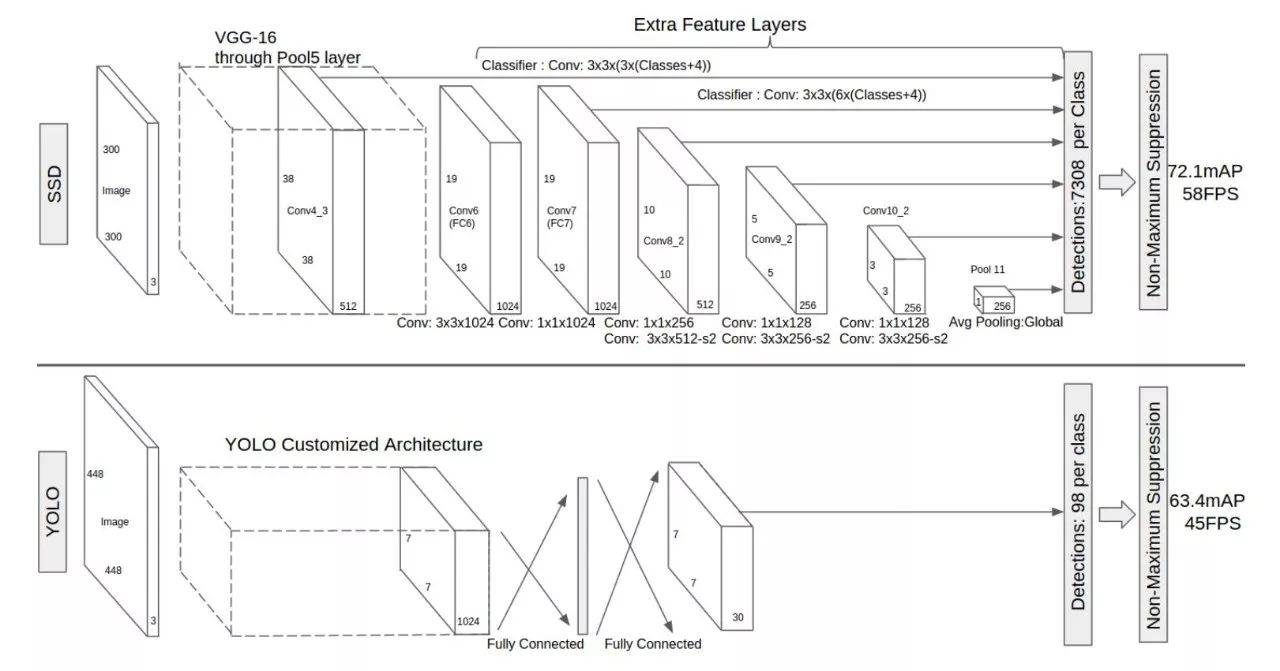• Mbox_loc
Conv4_3：$38\times38\times16$，Conv7_2：$19\times19\times24$，Conv8_2：$10\times10\times24$，Conv9_2：$5\times5\times24$，Conv10_2：$3\times3\times16$，Pool11：$1\times1\times16$
• Mbox_con
Conv4_3：$38\times38\times84$，Conv7_2：$19\times19\times126$，Conv8_2：$10\times10\times126$，Conv9_2：$5\times5\times126$，Conv10_2：$3\times3\times84$，Pool11：$1\times1\times84$

Mbox_loc的维度与特征图上每个点要预测的框的数量有关，六层特征图中每个点分别预测4，6，6，6，4，4个框，每个框需要4个值表达，所以预测为4时，channel为$4\times4\times=16$，预测为6时，channel为$4\times6\times=24$
Mbox_con的维度与SDD要预测类别置信度和框的数量有关，需要注意的是，SDD将背景类同样作为一个类别参与预测，所以当目标类别是0的时候，SDD实际要预测21个类别的置信度，所以当背景类的置信度最高时，也就意味着没有目标。同时，SDD要对每一个框都输出所有的置信度，这是SSD和YOLO不一样的地方，虽然YOLO的每一个格子也输出两个box，但是置信度确对应格子，而不是对应box， 但是SSD是对每个box都输出对应的置信度，所以当box的数量为4时，channel为$4\times21\times=81$，box的数量为6时，channel为$6\times21\times=126$

## SSD与RPN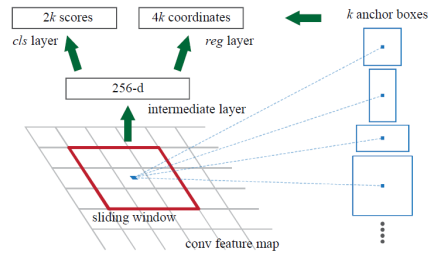## SSD与YOLO

SSD通过六个特征图，每个特征图上的点依次输出4，6，6，6，4，4个框，那么SDD一共可以有8732个预测框。
$38\times38\times4 + 19\times19\times6 + 10\times10\times6 + 5\times5\times6 + 3\times3\times6 + 1\times1\times16 = 8732$

## 先验框设定

• 设定目的
SSD的先验框是借鉴RPN，RPN中的anchor box有三种比例和三种尺度，这其实是在模拟实际图片中目标ground truth的尺寸和比例，并做一个合理的抽象，这使得预测框的初始值更贴近ground truth，也可以理解为，将原有的ground truth转化为ground truth与archor box的偏差，方便后续的优化训练。这里的反面例子就是YOLO，YOLO其实是一个没有archor的方法，所以YOLO在优化bbox的宽高时，是直接使用输出值逼近bbox宽高的归一化的值，这里是没有先验的从0开始，现对而言这个难度要比存在archor预设大一些。而SSD为了避免这一点，借鉴了RPN的archor预设。
• 如何设定
不同层输出的特征图，它们的感受野是不同的，一般情况下，随着下采样次数的增加，感受野在成倍数的放大。在这个前提下，如果用同一个规则设置不同层特征图的先验框是不合理的，所以SSD采用了一种线性规则：随着特征图层数的加深，特征图逐渐变小，先验框尺度线性增加。
$s_{k}=s_{min}+\frac{m_{max}-m_{min}}{m-1}(k-1),k\in[1,m]$
其中m指的特征图个数，但是$m=5$，而不是6，因为第一层（Conv4_3层）是单独设置的，$s_k$表示先验框大小相对于图片的比例，而$s_{min}$$s_{max}$表示比例的最小值与最大值，paper里面取0.2和0.9。对于第一个特征图，其先验框的尺度比例一般设置为0.1。所以特征图比例分别是0.1，0.2，0.375，0.55，0.725，0.9。这样对应$300\times300$的输入图像，计算出的尺寸就应该是30,60,112,165,217,270。
当然还有一组数字是30,60,111,162,213,264，这个是从SSD的caffe源码里求得的。当有当前层的尺度$s_{k}$和下一层的尺度$s_{k+1}$，SDD会根据这两个尺寸先计算两个大小不同，但长宽比都是1的正方形先验框，一个长宽是$s_{k}$，另一个长宽是$\sqrt {s_{k} \times s_{k+1}}$，需要注意的是，最后一个特征图的尺寸已经没有k+1了，所以这个值是推算出来的315。
尺寸确定之后，还剩下先验框的比例，这个使用的是$\left \{2,3,\frac{1}{2},\frac{1}{3}\right \}$这组数，都在尺寸为$s_{k}$的正方形框上产生，比如比例是2时，计算方式为：
$w=s_{k}\sqrt{2}$$h=\frac{s_{k}}{\sqrt{2}}$
当预设框是4个时，使用$\left \{2,\frac{1}{2}\right \}$，预设框是6个时，使用$\left \{2,3,\frac{1}{2},\frac{1}{3}\right \}$
• 如何使用
这些先验框最终被用于参与目标位置的计算，SSD要预测位置实际上是ground truth和预设框的offset，或者说，预设框被用于重新编码ground truth，这一点和Faster R-CNN很像，假设预设框为$\left \{d^{cx},d^{cy},d^{w},d^{h}\right \}$，ground truth为$\left \{g^{cx},g^{cy},g^{w},g^{h}\right \}$，从公式中可以看出来，$\left \{d^{cx},d^{cy}\right \}$$\left \{g^{cx},g^{cy}\right \}$都表示中心点坐标。那么转换后的ground truth坐标$\left \{\hat{g}^{cx},\hat{g}^{cy},\hat{g}^{w},\hat{g}^{h}\right \}$依照下面方式求取：
$\hat{g}^{cx}=\frac{\left (g^{cx}-d^{cx} \right )}{d^{w}}$$\hat{g}^{cy}=\frac{\left (g^{cy}-d^{cy} \right )}{d^{h}}$$\hat{g}^{w}=log{\frac{g_{w}}{d^{w}}}$$\hat{g}^{h}=log{\frac{g_{h}}{d^{h}}}$

# 损失函数

SSD的损失函数由两部分组成，分别是位置误差（locatization loss， loc）与置信度误差（confidence loss, conf）：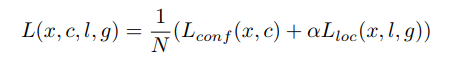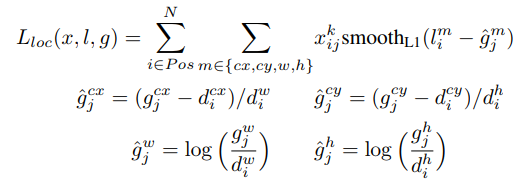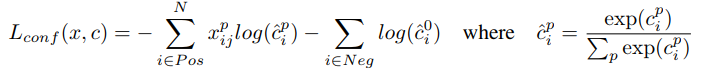# 性能评价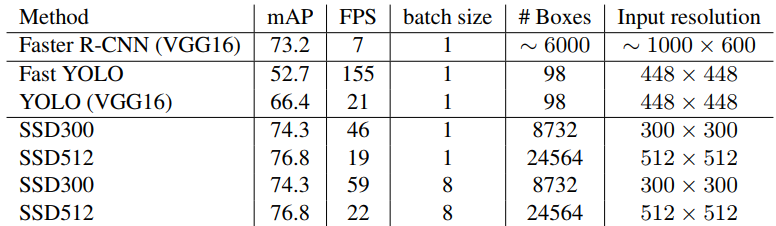• SSD为什么比YOLO还快
SSD的主干网络要比YOLO更大，因为它在VGG之后又加了很多层，同时SSD的分支全部靠卷积，而不是YOLO一样的reshape，那么为什么SSD的速度最后反而比YOLO小了呢？其实这个比较有一些取巧的地方，因为它们的输入图像不是一样大的，SSD300的输入图像只有$300\times300$，而YOLO是$448\times 448$，这为SSD节省下了很多算力。不过从两个角度考虑，$300\times300$分辨率的图像输入，mAP表现比$448\times 448$分辨率的图像输入还要好，这也说明了SSD算法确实提升非常大。同时YOLO也有更轻量的版本，FPS可以达到45，但是mAP就更低了，所以SDD并没有对比这个版本。
• SSD为什么对小目标同样不好
SSD的小目标校测效果，相比YOLO是有所改善的，因为扩张卷积增大感受野以及在特征图还比较大的时候，就拉取分支。但是相比于大的目标，SSD的小目标检测效果还是稍差一些，这是因为深层的特征图具有足够抽象的语义信息，这保证了目标类可以和背景类分开，但是特征图的尺寸确太小了；而浅层的特征图尺寸足够大，但是语义信息不够，不能保证目标类和背景类的区分。

09-22809

#### 目标检测（Object Detection）—— SSD总结04-165315

#### 使用object_detection_api进行训练和预测

11-274850

#### 一种新的特征融合方式：《Learning Spatial Fusion for Single-Shot Object Detection》解读

05-095万+

#### 目标检测（一）——目标检测综述（持续更新中）

09-2369

#### TensorFlow Object Detection API（Windows下训练）

01-16298

#### 目标检测系列（七）：SSD

02-04221

#### Tensorflow object detection api 详细实战教程（1）环境搭建与测试

03-22482

#### 目标检测算法（R-CNN，Fast R-CNN，Faster R-CNN，FPN，YOLOv1-v3）

01-172078

#### 【双语论文】Joint 3D Proposal Generation and Object Detection from View Aggregation©️2020 CSDN 皮肤主题: Age of Ai 设计师: meimeiellie点击重新获取扫码支付1.余额是钱包充值的虚拟货币，按照1:1的比例进行支付金额的抵扣。
2.余额无法直接购买下载，可以购买VIP、C币套餐、付费专栏及课程。余额充值